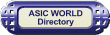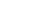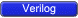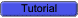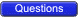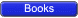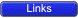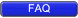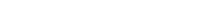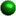Introduction

Verilog has built-in primitives like gates, transmission gates, and switches. This is a rather small number of primitives; if we need more complex primitives, then Verilog provides UDP, or simply User Defined Primitives. Using UDP we can model• Combinational Logic
• Sequential LogicWe can include timing information along with these UDP to model complete ASIC library models.Syntax

UDP begins with reserve word primitive and ends with endprimitive. Ports/terminals of primitive should follow. This is similar to what we do for module definition. UDPs should be defined outside module and endmodule```
1 //This code shows how input/output ports
2 // and primitve is declared
3 primitive udp_syntax (
4 a, // Port a
5 b, // Port b
6 c, // Port c
7 d  // Port d
8 );
9 output a;
10 input b,c,d;
11
12 // UDP function code here
13
14 endprimitive
```In the above code, udp_syntax is the primitive name, it contains ports a, b,c,d.The formal syntax of the UDP definition is as follows:```<UDP>
::= primitive <name_of_UDP> ( <output_terminal_name>,
<input_terminal_name> <,<input_terminal_name>>* ) ;
<UDP_declaration>+
<UDP_initial_statement>?
<table_definition>
endprimitive

<name_of_UDP>
::= <IDENTIFIER>

<UDP_declaration>
::= <UDP_output_declaration>
||= <reg_declaration>
||= <UDP_input_declaration>

<UDP_output_declaration>
::= output <output_terminal _name>;
<reg_declaration>
::=   reg <output_terminal_name> ;

<UDP_input_declaration>
::= input <input_terminal_name> <,<input_terminal_name>>* ;

<UDP_initial_statement>
::= initial <output_terminal_name> = <init_val> ;

<init_val>
::= 1'b0
||= 1'b1
||= 1'bx
||= 1
||= 0

<table_definition>
::= table
<table_entries>
endtable

<table_entries>
::= <combinational_entry>+
||= <sequential_entry>+

<combinational_entry>
::= <level_input_list> : <OUTPUT_SYMBOL> ;

<sequential_entry>
::= <input_list> : <state> : <next_state> ;

<input_list>
::= <level_input_list>
||= <edge_input_list>

<level_input_list>
::= <LEVEL_SYMBOL>+

<edge_input_list>
::= <LEVEL_SYMBOL>* <edge> <LEVEL_SYMBOL>*

<edge>
::= ( <LEVEL_SYMBOL> <LEVEL_SYMBOL> )
||= <EDGE_SYMBOL>

<state>
::= <LEVEL_SYMBOL>

<next_state>
::= <OUTPUT_SYMBOL>
||= -
```UDP ports rules• An UDP can contain only one output and up to 10 inputs.
• Output port should be the first port followed by one or more input ports.
• All UDP ports are scalar, i.e. Vector ports are not allowed.
• UDPs can not have bidirectional ports.
• The output terminal of a sequential UDP requires an additional declaration as type reg.
• It is illegal to declare a reg for the output terminal of a combinational UDPBody

Functionality of primitive (both combinational and sequential) is described inside a table, and it ends with reserved word 'endtable' as shown in the code below. For sequential UDP, we can use initial to assign an initial value to output.```
1 // This code shows how UDP body looks like
2 primitive udp_body (
3 a, // Port a
4 b, // Port b
5 c  // Port c
6 );
7 output a;
8 input b,c;
9
10 // UDP function code here
11 // A = B | C;
12 table
13  // B  C    : A
14     ?  1    : 1;
15     1  ?    : 1;
16     0  0    : 0;
17 endtable
18
19 endprimitive
```Note: An UDP cannot use 'z' in the input tableTestBench to check the above UDP

```
1 `include "udp_body.v"
2 module udp_body_tb();
3
4 reg b,c;
5 wire a;
6
7 udp_body udp (a,b,c);
8
9 initial begin
10   \$monitor(" B = %b C = %b  A = %b",b,c,a);
11   b = 0;
12   c = 0;
13    #1  b = 1;
14    #1  b = 0;
15    #1  c = 1;
16    #1  b = 1'bx;
17    #1  c = 0;
18    #1  b = 1;
19    #1  c = 1'bx;
20    #1  b = 0;
21    #1  \$finish;
22 end
23
24 endmodule
```Simulator Output```  B = 0 C = 0  A = 0
B = 1 C = 0  A = 1
B = 0 C = 0  A = 0
B = 0 C = 1  A = 1
B = x C = 1  A = 1
B = x C = 0  A = x
B = 1 C = 0  A = 1
B = 1 C = x  A = 1
B = 0 C = x  A = x
```Table

Table is used for describing the function of UDP. Verilog reserved word table marks the start of table and reserved word endtable marks the end of table.Each line inside a table is one condition; when an input changes, the input condition is matched and the output is evaluated to reflect the new change in input.Initial

Initial statement is used for initialization of sequential UDPs. This statement begins with the keyword 'initial'. The statement that follows must be an assignment statement that assigns a single bit literal value to the output terminal reg.```
1 primitive udp_initial (a,b,c);
2 output a;
3 input b,c;
4 reg a;
5 // a has value of 1 at start of sim
6 initial a = 1'b1;
7
8 table
9 // udp_initial behaviour
10 endtable
11
12 endprimitive
```Symbols

UDP uses special symbols to describe functions like rising edge, don't care and so on. The table below shows the symbols that are used in UDPs:Symbol Interpretation Explanation ? 0 or 1 or X ? means the variable can be 0 or 1 or x b 0 or 1 Same as ?, but x is not included f (10) Falling edge on an input r (01) Rising edge on an input p (01) or (0x) or (x1) or (1z) or (z1) Rising edge including x and z n (10) or (1x) or (x0) or (0z) or (z0) Falling edge including x and z * (??) All transitions - no change No ChangeCopyright © 1998-2014 Deepak Kumar Tala - All rights reserved Do you have any Comment? mail me at:deepak@asic-world.com